# Sum of members

What is the sum of the first two members of the aritmetic progression if d = -4.3 and a3 = 7.5?

Result

s =  27.9

#### Solution:Leave us a comment of example and its solution (i.e. if it is still somewhat unclear...):

Showing 0 comments:Be the first to comment!## Next similar examples:

1. Difference AP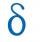Calculate the difference of arithmetic progression if the sum of its first 19 members Sn = 8075 and the first member is a1 = 20
2. SequenceWrite the first 7 members of an arithmetic sequence: a1=-3, d=6.
3. SequenceWrite the first 6 members of these sequence: a1 = 5 a2 = 7 an+2 = an+1 +2 an
4. Sequence 2Write the first 5 members of an arithmetic sequence a11=-14, d=-1
5. Sequence 3Write the first 5 members of an arithmetic sequence: a4=-35, a11=-105.
6. SequenceBetween numbers 1 and 53 insert n members of the arithmetic sequence that its sum is 702.
7. AS sequenceIn an arithmetic sequence is given the difference d = -3 and a71 = 455. a) Determine the value of a62 b) Determine the sum of 71 members.
8. Theorem proveWe want to prove the sentense: If the natural number n is divisible by six, then n is divisible by three. From what assumption we started?
9. Saving per cents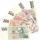The first day I save 1 cent and every next day cent more. How many I saved per year (365 days)?
10. Consecutive numbersSum of ten consecutive numbers is 105. Determine these numbers (write first and last).
11. Apples in baskets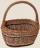Determine how many apples are in baskets when in the first basket are 4 apples, and in any other is 29 apples more than the previous, and we have eight baskets.
12. SeatsSeats in the sport hall are organized so that each subsequent row has five more seats. First has 10 seats. How many seats are: a) in the eighth row b) in the eighteenth row
13. AP - simpleDetermine the first nine elements of sequence if a10 = -1 and d = 4
14. 6 termsFind the first six terms of the sequence. a1 = 7, an = an-1 + 6
15. Nineteenth memberFind the nineteenth member of the arithmetic sequence: a1=33 d=5 find a19
16. Series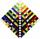Your task is express the sum of the following arithmetic series for n = 14: S(n) = 11 + 13 + 15 + 17 + ... + 2n+9 + 2n+11
17. Vectors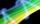For vector w is true: w = 2u-5v. Determine coordinates of vector w if u=(3, -1), v=(12, -10)